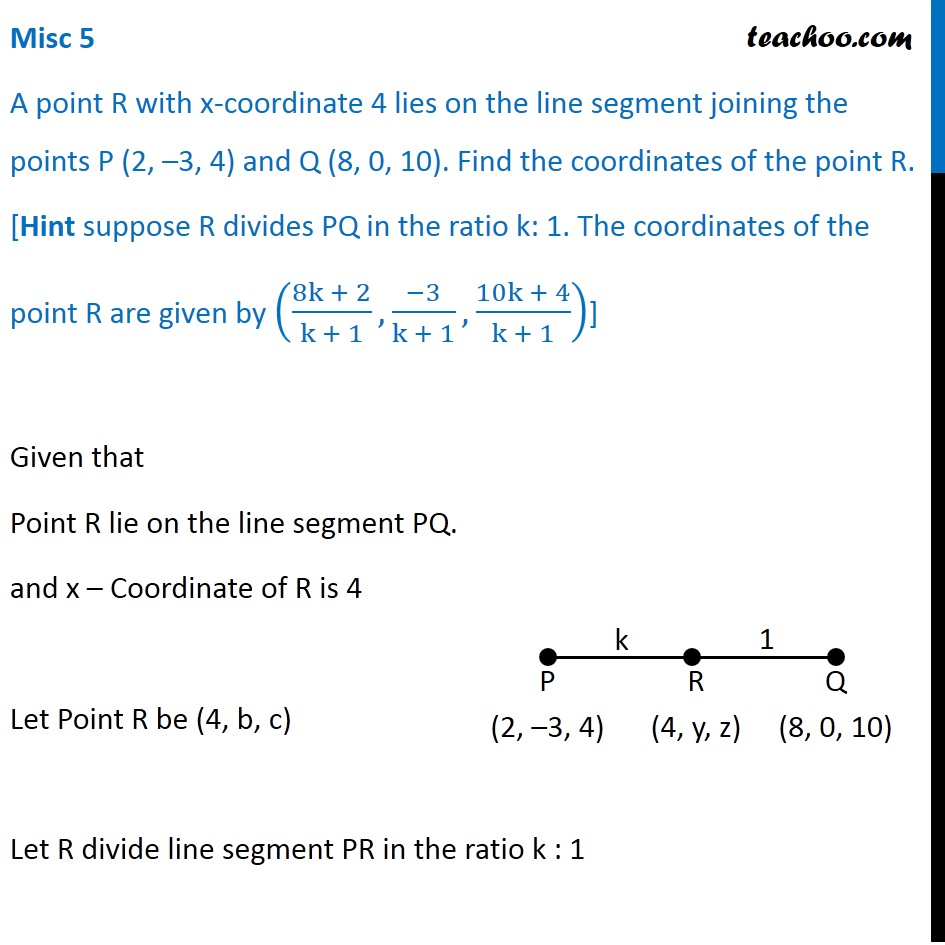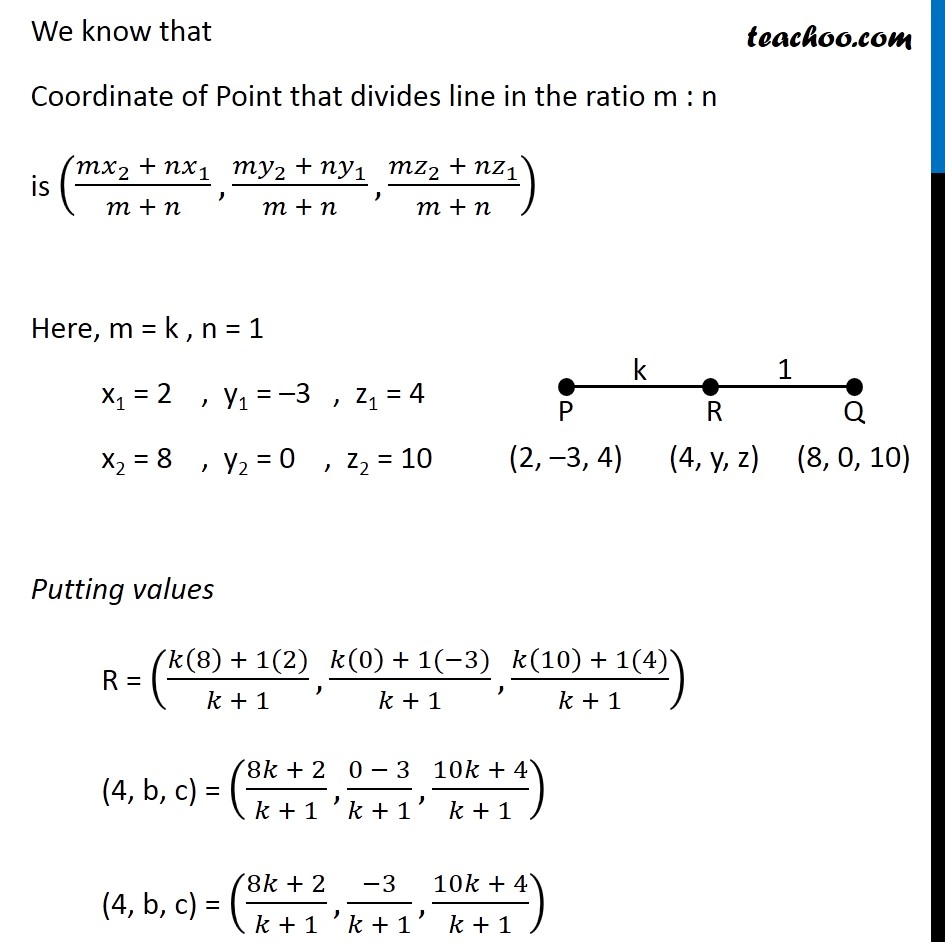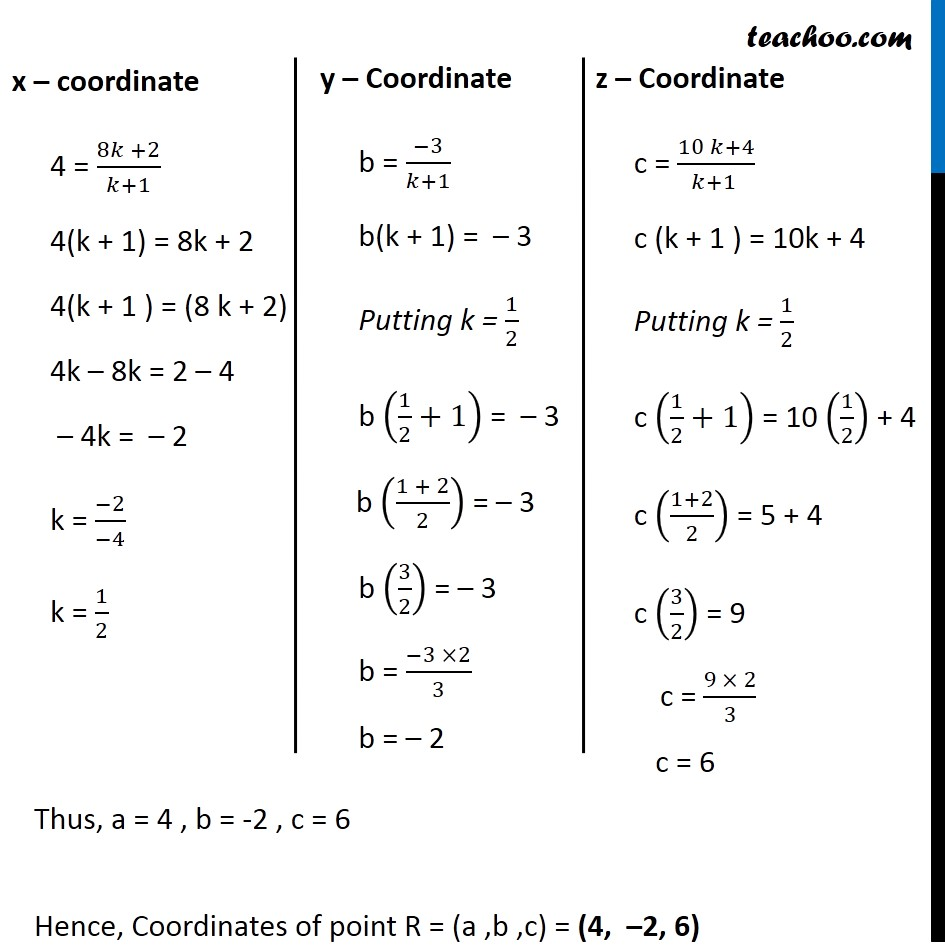Miscellaneous

Chapter 11 Class 11 - Intro to Three Dimensional Geometry
Serial order wiseLearn in your speed, with individual attention - Teachoo Maths 1-on-1 Class

### Transcript

Misc 5 A point R with x-coordinate 4 lies on the line segment joining the points P (2, –3, 4) and Q (8, 0, 10). Find the coordinates of the point R. [Hint suppose R divides PQ in the ratio k: 1. The coordinates of the point R are given by ((8k + 2)/(k + 1),(−3)/(k + 1),(10k + 4)/(k + 1))] Given that Point R lie on the line segment PQ. and x – Coordinate of R is 4 Let Point R be (4, b, c) Let R divide line segment PR in the ratio k : 1 We know that Coordinate of Point that divides line in the ratio m : n is ((〖𝑚𝑥〗_2 + 〖𝑛𝑥〗_1)/(𝑚 + 𝑛),(〖𝑚𝑦〗_2 + 〖𝑛𝑦〗_1)/(𝑚 + 𝑛),(〖𝑚𝑧〗_2 + 〖𝑛𝑧〗_1)/(𝑚 + 𝑛)) Here, m = k , n = 1 x1 = 2 , y1 = –3 , z1 = 4 x2 = 8 , y2 = 0 , z2 = 10 Putting values R = ((𝑘(8) + 1(2))/(𝑘 + 1),(𝑘(0) + 1(−3))/(𝑘 + 1),(𝑘(10) + 1(4))/(𝑘 + 1)) (4, b, c) = ((8𝑘 + 2)/(𝑘 + 1),(0 − 3)/(𝑘 + 1),(10𝑘 + 4)/(𝑘 + 1)) (4, b, c) = ((8𝑘 + 2)/(𝑘 + 1),(−3)/(𝑘 + 1),(10𝑘 + 4)/(𝑘 + 1)) x – coordinate 4 = (8𝑘 +2)/(𝑘+1) 4(k + 1) = 8k + 2 4(k + 1 ) = (8 k + 2) 4k – 8k = 2 – 4 – 4k = – 2 k = (−2)/(−4) k = 1/2 y – Coordinate b = (−3)/(𝑘+1) b(k + 1) = – 3 Putting k = 1/2 b (1/2+1) = – 3 b ((1 + 2)/2) = – 3 b (3/2) = – 3 b = (−3 ×2)/3 b = – 2 z – Coordinate c = (10 𝑘+4)/(𝑘+1 ) c (k + 1 ) = 10k + 4 Putting k = 1/2 c (1/2+1) = 10 (1/2) + 4 c ((1+2)/2) = 5 + 4 c (3/2) = 9 c = (9 × 2)/3 c = 6 Thus, a = 4 , b = -2 , c = 6 Hence, Coordinates of point R = (a ,b ,c) = (4, –2, 6) Question 2 A point R with x-coordinate 4 lies on the line segment joining the points P (2, 3, 4) and Q (8, 0, 10). Find the coordinates of the point R. [Hint suppose R divides PQ in the ratio k: 1. The coordinates of the point R are given by 8k+2 k+1 , 3 k+1 , 10k+4 k+1 ] Given that Point R lie on the line segment PQ. and x Coordinate of R is 4 Let Point R be (4, b, c) Let R divide line segment PR in the ratio k : 1 We know that Coordinate of Point that divide line segment joining (x1 y1 z1) & (x2 y2 z2) in the ratio m : n is 2 + 1 + , 2 + 1 + , 2 + 1 + Here, m = k , n = 1 x1 = 2 , y1 = 3 , x2 = 8 , y2 = 0 Putting values R = 8 +1(2) +1 , 0 +1( 3) +1 , 10 +1(4) +1 (4, b, c) = 8 +2 +1 , 0 3 +1 , 10 +4 +1 (4, b, c) = 8 +2 +1 , 3 +1 , 10 +4 +1